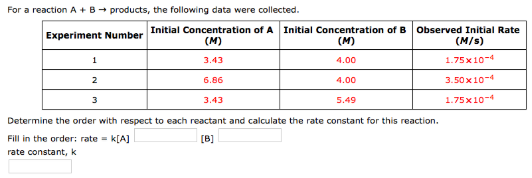# Problem: For a reaction A + B → products, the following data were collected. Determine the order with respect to each reactant and calculate the rate constant for this reaction. Fill in the order: rate = k[A] __________ [B] ____________rate constant, k ____________

###### FREE Expert Solution
80% (341 ratings)###### Problem Details

For a reaction A + B → products, the following data were collected.

Determine the order with respect to each reactant and calculate the rate constant for this reaction.

Fill in the order: rate = k[A] __________ [B] ____________

rate constant, k ____________